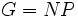# Gamma group

This article defines a group property: a property that can be evaluated to true/false for any given group, invariant under isomorphism
View a complete list of group properties
VIEW RELATED: Group property implications | Group property non-implications |Group metaproperty satisfactions | Group metaproperty dissatisfactions | Group property satisfactions | Group property dissatisfactions

## Contents

This article defines a term that has been used or referenced in a journal article or standard publication, but may not be generally accepted by the mathematical community as a standard term.[SHOW MORE]

## Definition

### Definition with symbols

A finite non-Abelian group$G$ is termed a$\Gamma$-group if$G = NP$ where$N$ is a normal elementary Abelian 2-subgroup and$P$ is a cyclic group of prime order acting irreducibly on$N$ (in other words, no proper subgroup of$N$ is$P$-invariant).